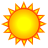# Calculating percentage of a polygon's area and using it to buffer a polyline

170
1
01-30-2012 02:10 PMNew Contributor
Hi all,

I've got a tricky one (or maybe not!). I've been asked to calculate the percentage of a series of polygons' area, and then distribute that resulting area within each polygon, but along the features of a polyline file. In this case, it would be 15% of the total area of the polygon. My first idea was to use the buffer by field option to calculate the buffer area for each polygon (which would solve the first have of the problem), but I kept getting an empty output area error (still not sure why).

The particular example I'm working with is a series of parcels (the polygons) and a roads file (the polyline), where we're hoping to be able to allocate 25% of each parcel to a specific purpose along the roads.

Thanks!
Tags (3)Regular Contributor III
Know any Python scripting?

I can think of a way to do thins using an iterative trial-and-error sort of thing that would seek to "get close" to the 25% goal. A possible algirithm might lolok like this:

1. Extract a single parcel
2. Buffer the road segment next to the parcel by 10feet (something small) and then 50ft (something larger)
3. Get the % overlap of each of those buffer distances - that is maybe the 10ft buffer covers 15% of the parcel and the 50ft buffer covers 75% of the parcel. Use Intersect tool to get the % overlap.
4. Using some basic linear interpolation (when y = 10 then x = .15, and when y = 50 then x = .75) solve for when x = .25. The script below might help.
5. Step 4 should get you close, and using some looping, you could then further refine the buffer distance so that you get to within some acceptabe tolerance of the 25% goal.
6. Then move on to the next parcel, rinse and repeat.

```def findLinearY(x1,y1,x2,y2,xValue):
#using the linear form of y = mx + b, solve for y...
slope = (y1 - y2) / float(x1 - x2)
b = y1 - (slope * x1)
return slope * float(xValue) + b #return a floating point mx + b!```

So, for example:

>>> print findLinear(0,0,5,5,2)
2.0
>>> print findLinearY(0,0,1,2,500.3)
1000.6